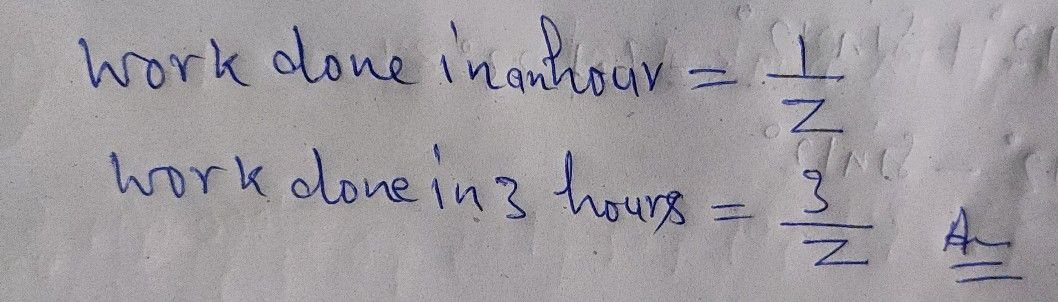Symbol
Problem$0ct1on6$ Read each question carefully. Write the letter that corresponds to your $an5y4c$ $011$ separate sheet of $.11001$ Write the given verbal phrase $|$ $0a1110ma11ca|y$ part of the work done $111$ $1$ hours $1$ $1110$ $1$ $y01r$ $1.111$ be done in $2$ hours $1$
7th-9th grade
Algebra
Search count: 106
SolutionQanda teacher - Ranjeetif you have no query regarding this question ,please evaluate the answer with your review